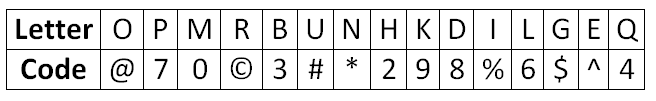# Alphanumeric Series

• Home -
• Alphanumeric Series

Alphanumeric Series - Topic Wise Multiple Choice Questions (MCQ)

Online Topic Wise Alphanumeric Series MCQ for competitive exam Practice Multiple Choice Questions for IBPS SSC Online Test Private Job Interview

1 )

L F 3 # R N 8 A @ Y 4 M © W P 6 H U 9 I K 2 E

Q . If all numbers are dropped from the above arrangement, which of the following will be the fourteenth from the left end?

I
P
W
U
Y

View Solution

2 )

In question below is given a group of letters followed by four combinations of digits/symbols given in (A), (B), (C) and (D). You have to find out which of the combinations correctly represents the group of digits based on the rules and mark the answer accordingly. If none of the combinations correctly represents the group of letters, mark (E) as your answer.Que:    UEPMOQ

3^70@#
4^70!#
4^70@#
None of these

View Solution

3 )

In question below is given a group of letters followed by four combinations of digits/symbols given in (A), (B), (C) and (D). You have to find out which of the combinations correctly represents the group of digits based on the rules and mark the answer accordingly. If none of the combinations correctly represents the group of letters, mark (E) as your answer.Que:   OPHDLU

#@286#
@#286#
@#246
@#286
None of these

View Solution

4 )

In question below is given a group of letters followed by four combinations of digits/symbols given in (A), (B), (C) and (D). You have to find out which of the combinations correctly represents the group of digits based on the rules and mark the answer accordingly. If none of the combinations correctly represents the group of letters, mark (E) as your answer.Que:   MIRKNQ

None of these

View Solution

5 )

In question below is given a group of letters followed by four combinations of digits/symbols given in (A), (B), (C) and (D). You have to find out which of the combinations correctly represents the group of digits based on the rules and mark the answer accordingly. If none of the combinations correctly represents the group of letters, mark (E) as your answer.Que:    HOLUIB

26@!%3
26^#%3
26@#%7
24@#%3
None of these

View Solution